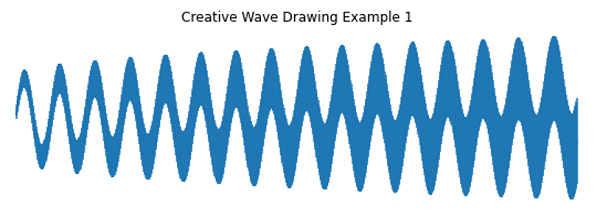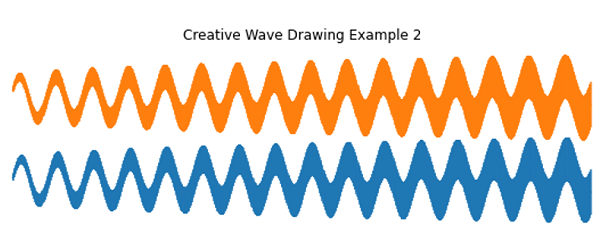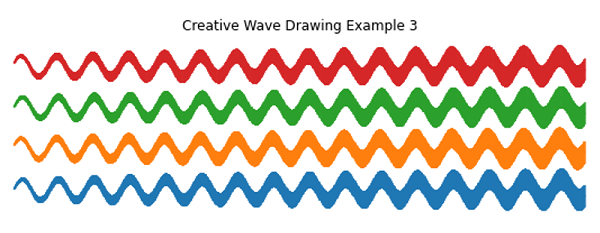In this article, we are going to create wave design using matplotlib in Python plotting.
Submitted by Anuj Singh, on August 21, 2020

Apart from scientific and data plotting, python can be used for drawing creative designs with finer constructions. In this example, we have drawn wave designs (three examples) using the feature of plotting error bars in matplotlib. Errorbar helped us to plot waves with a consistent increase in width of waves which could be a problem designing this wave in other platforms. Since we are using mathematical functions at the core, it is very fine and definitive.

Illustrations:```import numpy as np
import matplotlib.pyplot as plt

x = np.arange(0.1, 100, 0.1)
y = np.sin(x)
y2 = 4+ np.cos(x+5)

yerr = 0.1 + 0.1 * np.sqrt(x)

plt.figure(figsize=(10,3))
plt.errorbar(x, y, yerr=yerr)
plt.axis(False)
plt.title('Creative Wave Drawing Example 1')
plt.show()

plt.figure(figsize=(10,3))
plt.errorbar(x, y, yerr=yerr)
plt.errorbar(x, y2, yerr=yerr)
plt.axis(False)
plt.title('Creative Wave Drawing Example 2')
plt.show()

yy = 8 + np.sin(x)
y3 = 12 + np.cos(x+5)

plt.figure(figsize=(10,3))
plt.errorbar(x, y, yerr=yerr)
plt.errorbar(x, y2, yerr=yerr)
plt.errorbar(x, yy, yerr=yerr)
plt.errorbar(x, y3, yerr=yerr)
plt.axis(False)
plt.title('Creative Wave Drawing Example 3')
plt.show()
```

Output:

```Output is as Figure
```

Languages: » C » C++ » C++ STL » Java » Data Structure » C#.Net » Android » Kotlin » SQL
Web Technologies: » PHP » Python » JavaScript » CSS » Ajax » Node.js » Web programming/HTML
Solved programs: » C » C++ » DS » Java » C#
Aptitude que. & ans.: » C » C++ » Java » DBMS
Interview que. & ans.: » C » Embedded C » Java » SEO » HR
CS Subjects: » CS Basics » O.S. » Networks » DBMS » Embedded Systems » Cloud Computing
» Machine learning » CS Organizations » Linux » DOS
More: » Articles » Puzzles » News/Updates